# C++ Programming Quick Assignment: Please make sure ALL parts are answered, the code compiles and runs for a thumbs up :-)

C++ Programming Quick Assignment: Please make sure ALL parts are answered, the code compiles and runs for a thumbs up 🙂

Programming Exercise 1 The genetic information encoded in a strand of deoxyribonucleic acid (DNA) is stored in the purine and pyrimidine bases (adenine, guanine, cytosine, and thymine) that form the strand. Biologists are keenly interested in the bases in a DNA sequence because these bases determine what the sequence does. By convention DNA sequences are represented by using lists containing the letters “A” “G”, “C”, and “T” or adenine, guanine cytosine, and thymine, respectively). The following function computes one property of a DNA sequence-the number of times each base occurs in the sequence.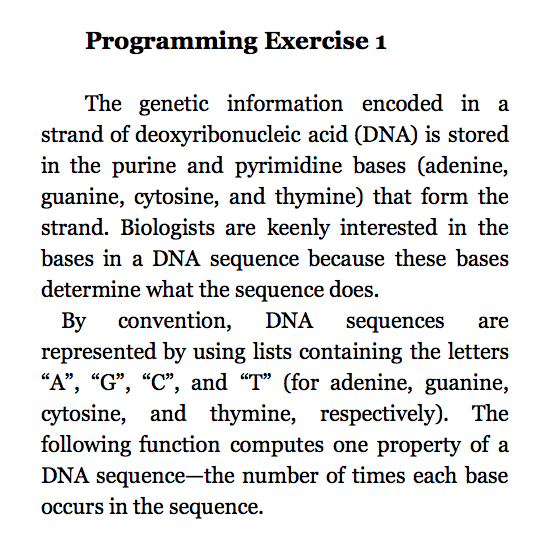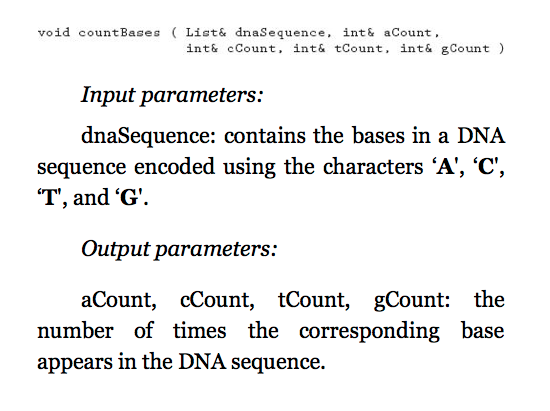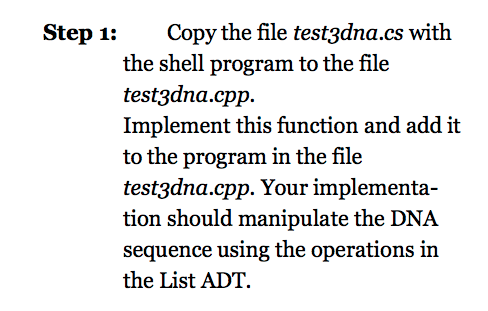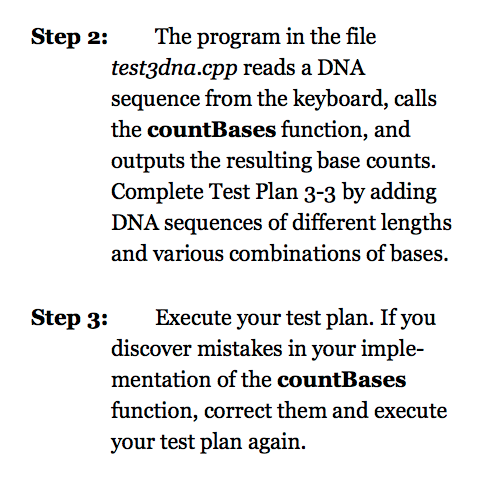config.h:

/**

* List class (Lab 3/Lab 4) configuration file.

* Activate test #N by defining the corresponding LAB3_TESTN to have the value 1.

*

* Because you will copy the List class code to your ordered list directory, having

* two “config.h” files presented the risk of accidentally replacing the one in the

* ordered list directory. So the two configuration files are combined for labs 3 and 4.

*

* NOTE!!! There was an error in the printed book. TEST1 shows up twice in the book.

* The basic List implementation uses TEST1 as described below, then exercise 2

* is activated by TEST2

*/

#define LAB3_TEST1 0 // 0 => test with char, 1 => test with int

#define LAB3_TEST2 0 // Prog exercise 2: moveToNth

#define LAB3_TEST3 0 // Prog exercise 3: find

/**

* Ordered list class tests.

*/

#define LAB4_TEST1 0 // merge: programming exercise 2

#define LAB4_TEST2 0 // subset: programming exercise 3

ListArray.h:

//——————————————————————–

//

// Laboratory 3                                           ListArray.h

// **Instructor’s Solution**

// Class declaration for the array implementation of the List ADT

//

//——————————————————————–

#ifndef LISTARRAY_H

#define LISTARRAY_H

#include <stdexcept>

#include <iostream>

using namespace std;

#pragma warning( disable : 4290 )

template < typename DataType >

class List

{

public:

static const int MAX_LIST_SIZE = 10;   // Default maximum list size

// Constructors

List ( int maxNumber = MAX_LIST_SIZE ); // Default constructor

List ( const List& source ); // Copy constructor

List& operator= ( const List& source );

// Destructor

virtual ~List ();

// List manipulation operations

virtual void insert ( const DataType& newDataItem ) // Insert after cursor

throw ( logic_error );

void remove () throw ( logic_error );        // Remove data item

virtual void replace ( const DataType& newDataItem ) // Replace data item

throw ( logic_error );

void clear ();                               // Clear list

// List status operations

bool isEmpty () const;                    // List is empty

bool isFull () const;                     // List is full

// List iteration operations

void gotoBeginning ()                     // Go to beginning

throw ( logic_error );

void gotoEnd ()                           // Go to end

throw ( logic_error );

bool gotoNext ()                          // Go to next data item

throw ( logic_error );

bool gotoPrior ()                         // Go to prior data item

throw ( logic_error );

DataType getCursor () const

throw ( logic_error );                // Return data item

// Output the list structure — used in testing/debugging

virtual void showStructure () const;

// In-lab operations

void moveToNth ( int n )                  // Move data item to pos. n

throw ( logic_error );

bool find ( const DataType& searchDataItem )     // Find data item

throw ( logic_error );

protected:

// Data members

int maxSize,

size,             // Actual number of data item in the list

cursor;           // Cursor array index

DataType *dataItems; // Array containing the list data item

};

#endif

ListArray.cpp:

#include “ListArray.h”

template < typename DataType >

List<DataType>::List ( int maxNumber )

{

}

template < typename DataType >

List<DataType>::List ( const List& source )

{

}

template < typename DataType >

List<DataType>& List<DataType>::operator= ( const List& source )

{

return *this;

}

template < typename DataType >

List<DataType>::~List ()

{

}

template < typename DataType >

void List<DataType>::insert ( const DataType& newDataItem )

throw ( logic_error )

{

}

template < typename DataType >

void List<DataType>::remove () throw ( logic_error )

{

}

template < typename DataType >

void List<DataType>::replace ( const DataType& newDataItem )

throw ( logic_error )

{

}

template < typename DataType >

void List<DataType>::clear ()

{

}

template < typename DataType >

bool List<DataType>::isEmpty () const

{

return false;

}

template < typename DataType >

bool List<DataType>::isFull () const

{

return false;

}

template < typename DataType >

void List<DataType>::gotoBeginning ()

throw ( logic_error )

{

}

template < typename DataType >

void List<DataType>::gotoEnd ()

throw ( logic_error )

{

}

template < typename DataType >

bool List<DataType>::gotoNext ()

throw ( logic_error )

{

return false;

}

template < typename DataType >

bool List<DataType>::gotoPrior ()

throw ( logic_error )

{

return false;

}

template < typename DataType >

DataType List<DataType>::getCursor () const

throw ( logic_error )

{

DataType t;

return t;

}

#include “show3.cpp”

template < typename DataType >

void List<DataType>::moveToNth ( int n )

throw ( logic_error )

{

}

template < typename DataType >

bool List<DataType>::find ( const DataType& searchDataItem )

throw ( logic_error )

{

return false;

}

show3.cpp:

//——————————————————————–

//

// Laboratory 3                                          show3.cpp

//

// Array implementation of the showStructure operation for the

//

//——————————————————————–

#include “ListArray.h”

template <typename DataType>

void List<DataType>:: showStructure () const

// outputs the data items in a list. if the list is empty, outputs

// “empty list”. this operation is intended for testing/debugging

// purposes only.

{

int j;   // loop counter

if ( size == 0 )

cout << “empty list” << endl;

// The Ordered List code blows up below. Since this is just debugging

// code, we check for whether the OrderedList is defined, and if so,

// print out the key value. If not, we try printing out the entire item.

// Note: This assumes that you have used the double-inclusion protection

// in your OrderedList.cpp file by doing a “#ifndef ORDEREDLIST_CPP”, etc.

// If not, you will need to comment out the code in the section under

// the “else”, otherwise the compiler will go crazy in lab 4.

// The alternative is to overload operator<< for all data types used in

// the ordered list.

else

{

cout << “size = ” << size

<< ”   cursor = ” << cursor << endl;

for ( j = 0 ; j < maxSize ; j++ )

cout << j << “t”;

cout << endl;

for ( j = 0 ; j < size ; j++ ) {

if( j == cursor ) {

cout << “[“;

cout << dataItems[j]

#ifdef ORDEREDLIST_CPP

.getKey()

#endif

;

cout << “]”;

cout << “t”;

}

else

cout << dataItems[j]

#ifdef ORDEREDLIST_CPP

.getKey()

#endif

<< “t”;

}

cout << endl;

}

}

test3dna.cpp:

//——————————————————————–

//

// Laboratory 3, In-lab Exercise 1                     test3dna.cpp

//

// Test program for the countbases function

//

//——————————————————————–

// Reads a DNA sequence from the keyboard, calls function countBases

// countBases (which uses a list to represent a DNA sequence), and

// outputs the number of times that each base (A, G, C and T) occurs

// in the sequence.

#include <iostream>

#include “ListArray.cpp”

using namespace std;

//——————————————————————–

//

// Function prototype

//

void countBases ( List<char> &dnaSequence,

int &aCount,

int &cCount,

int &tCount,

int &gCount              );

//——————————————————————–

int main ()

{

List<char> dnaSequence(25);   // DNA sequence (25 bases max.)

char base;              // DNA base

int aCount,             // Number of A’s in the sequence

cCount,             // Number of C’s in the sequence

tCount,             // Number of T’s in the sequence

gCount;             // Number of G’s in the sequence

// Read the DNA sequence from the keyboard.

cout << endl << “Enter a DNA sequence: “;

cin.get(base);

while ( base != ‘n’ )

{

dnaSequence.insert(base);

cin.get(base);

}

// Display the sequence.

cout << “Sequence: “;

if( dnaSequence.isEmpty() )

cout << “list is empty” << endl;

else

{

dnaSequence.gotoBeginning();

do

{

cout << dnaSequence.getCursor() << ” “;

} while ( dnaSequence.gotoNext() );

cout << endl;

}

// Count the number of times that each base occurs.

countBases(dnaSequence,aCount,cCount,tCount,gCount);

// Output the totals.

cout << “Number of A’s : ” << aCount << endl;

cout << “Number of C’s : ” << cCount << endl;

cout << “Number of T’s : ” << tCount << endl;

cout << “Number of G’s : ” << gCount << endl;

}

//——————————————————————–

//

// Insert your countBases function below.

//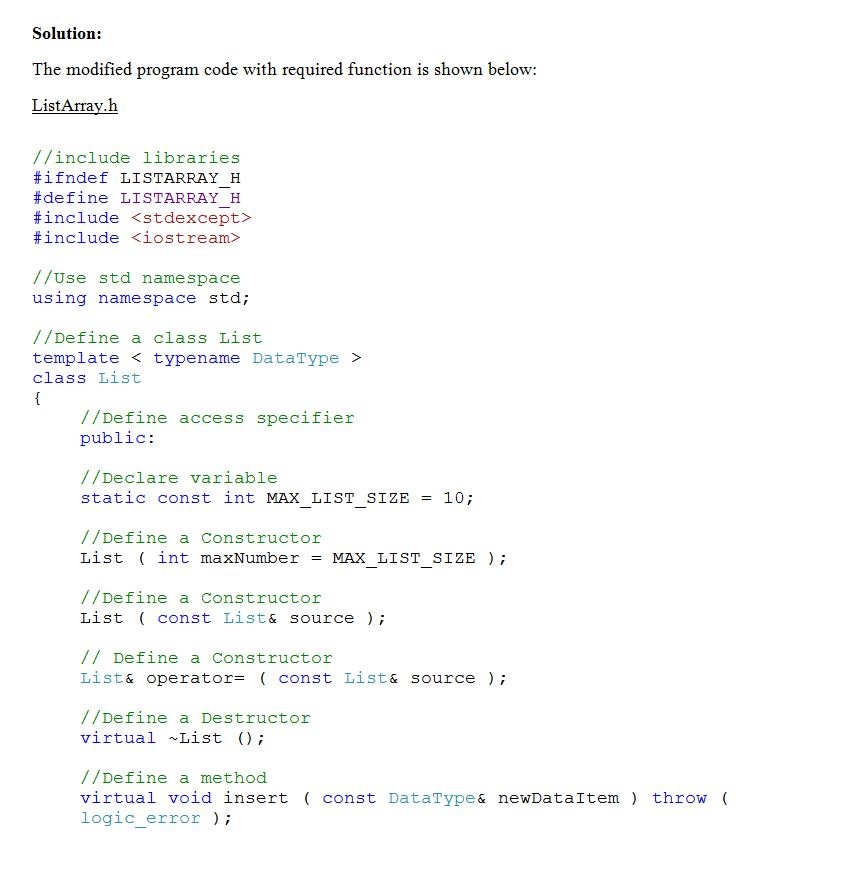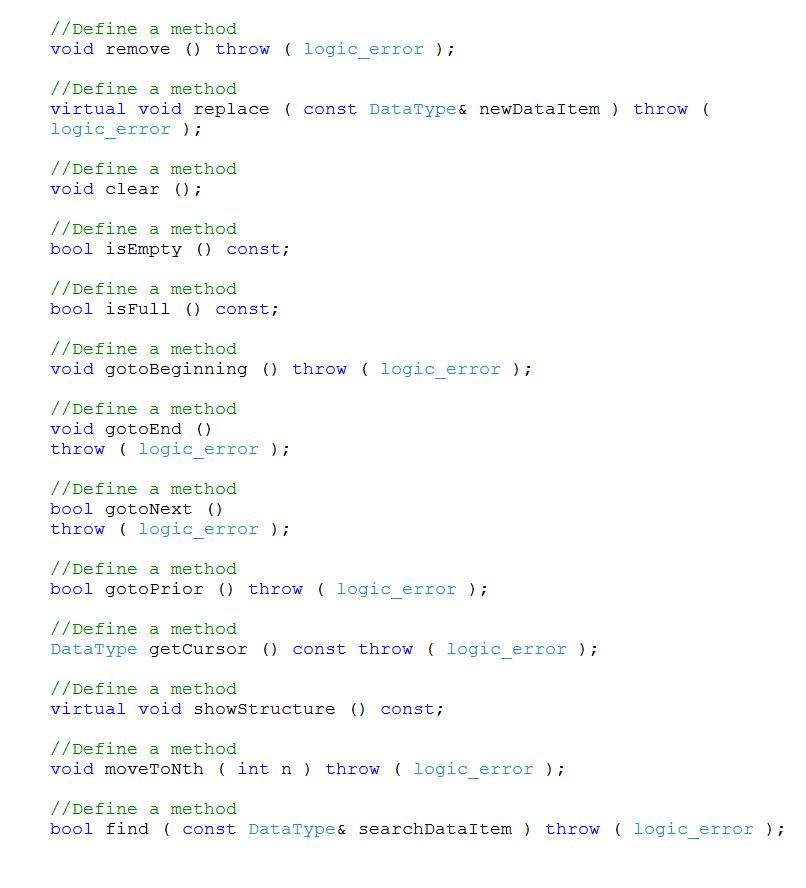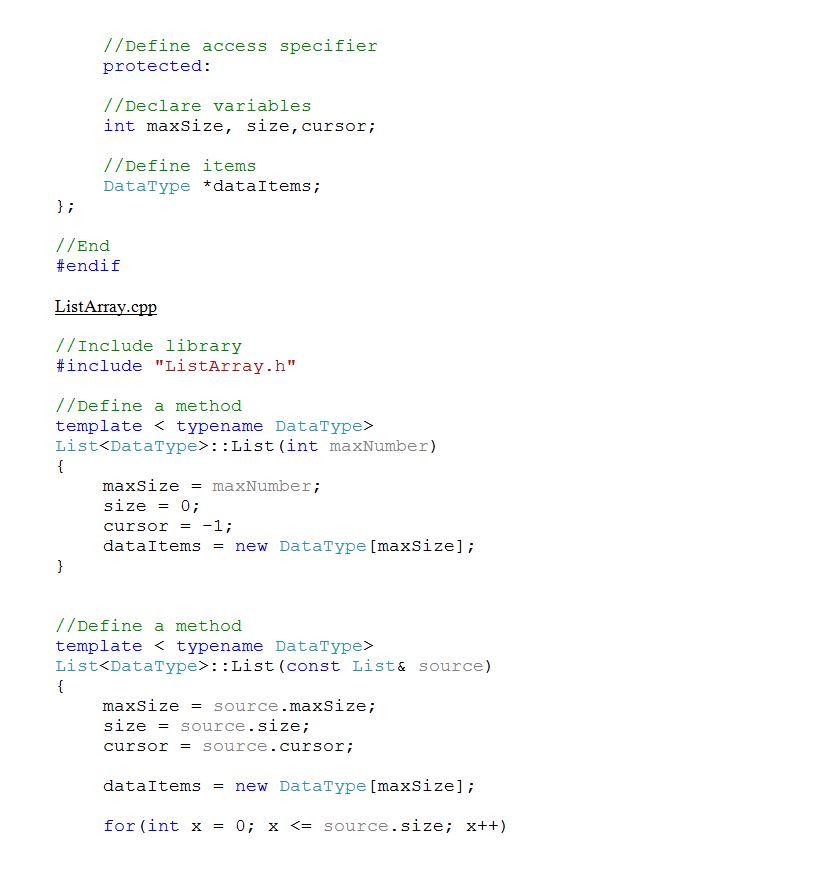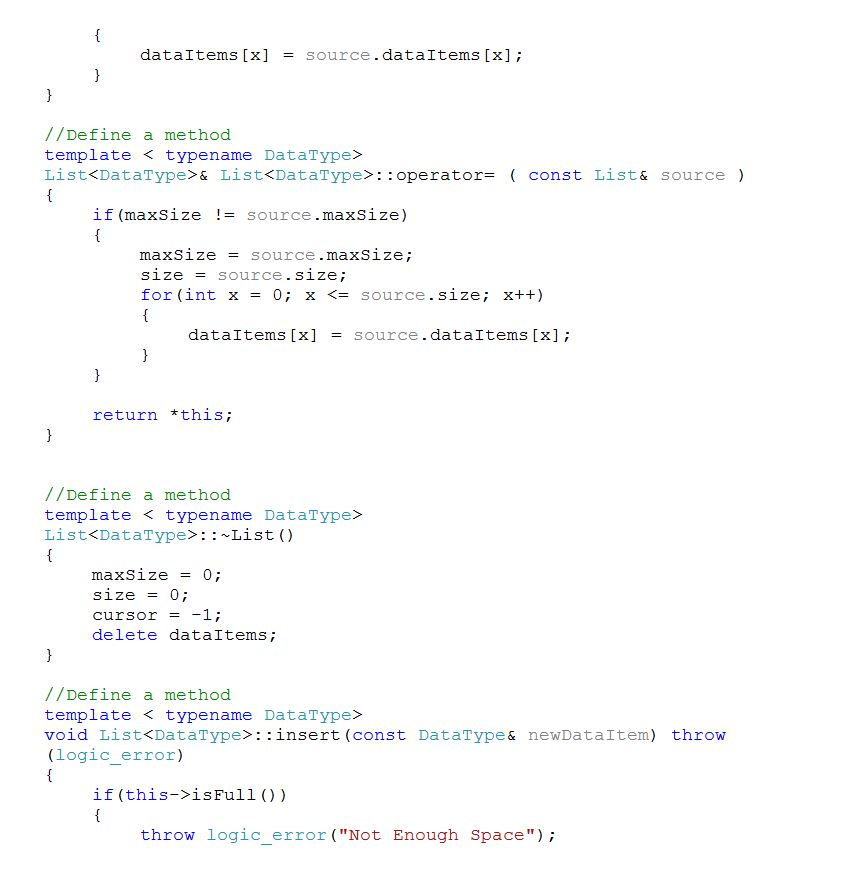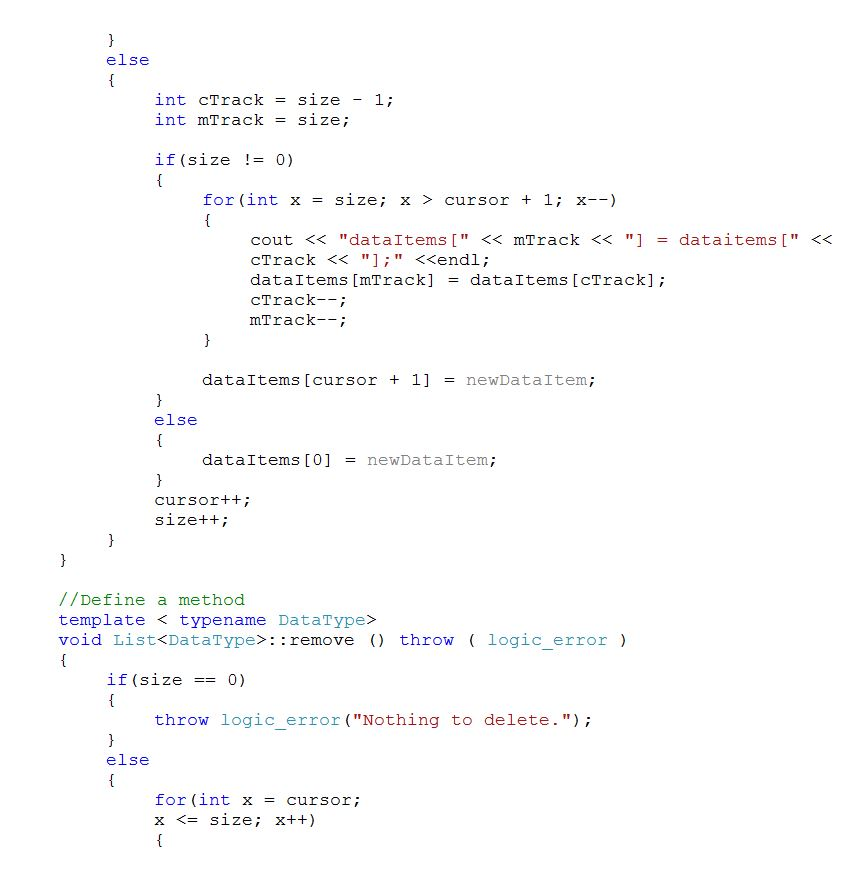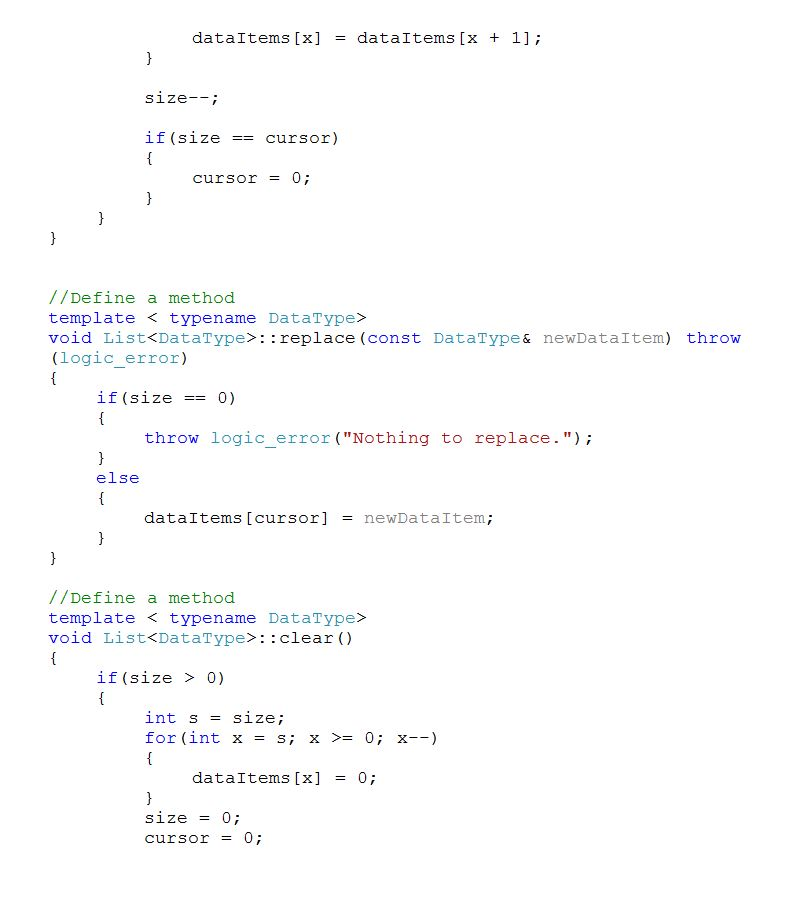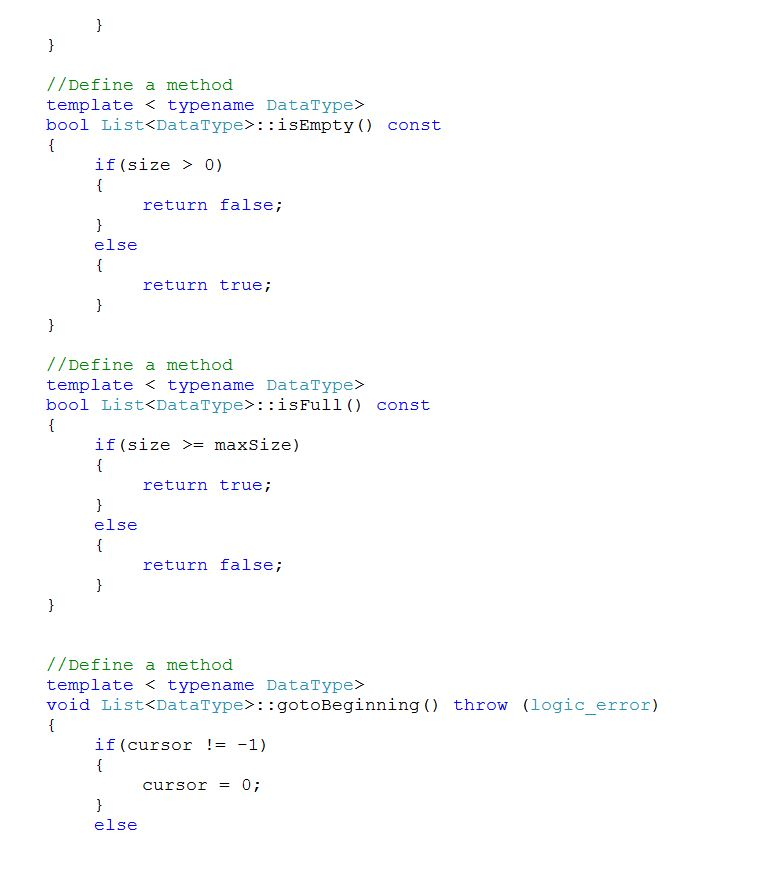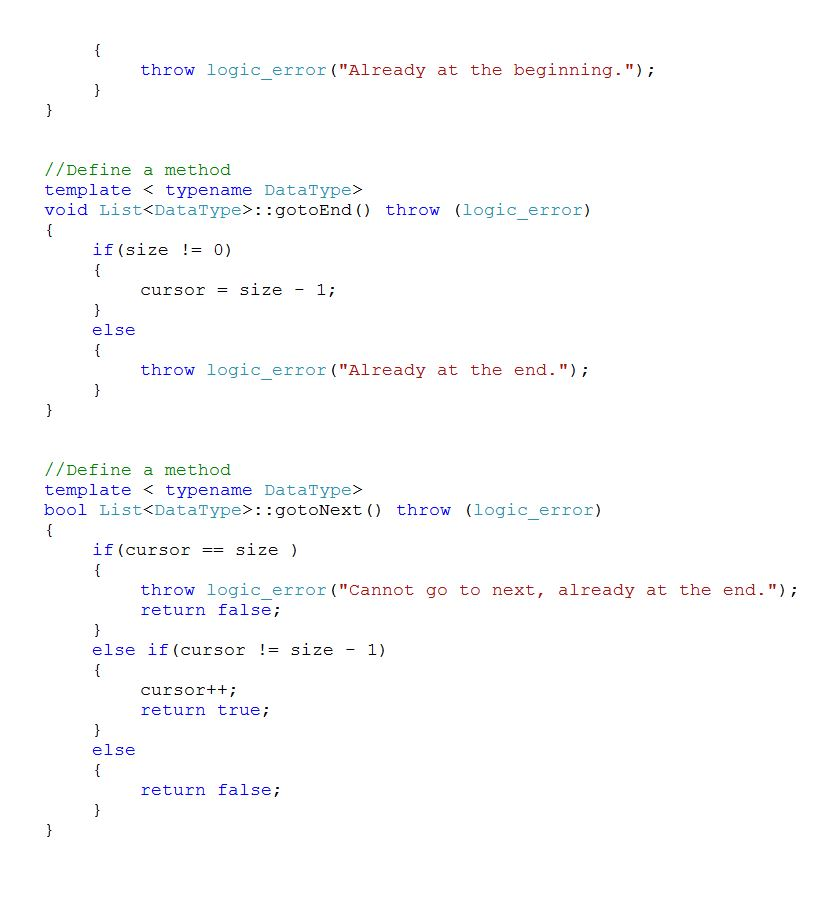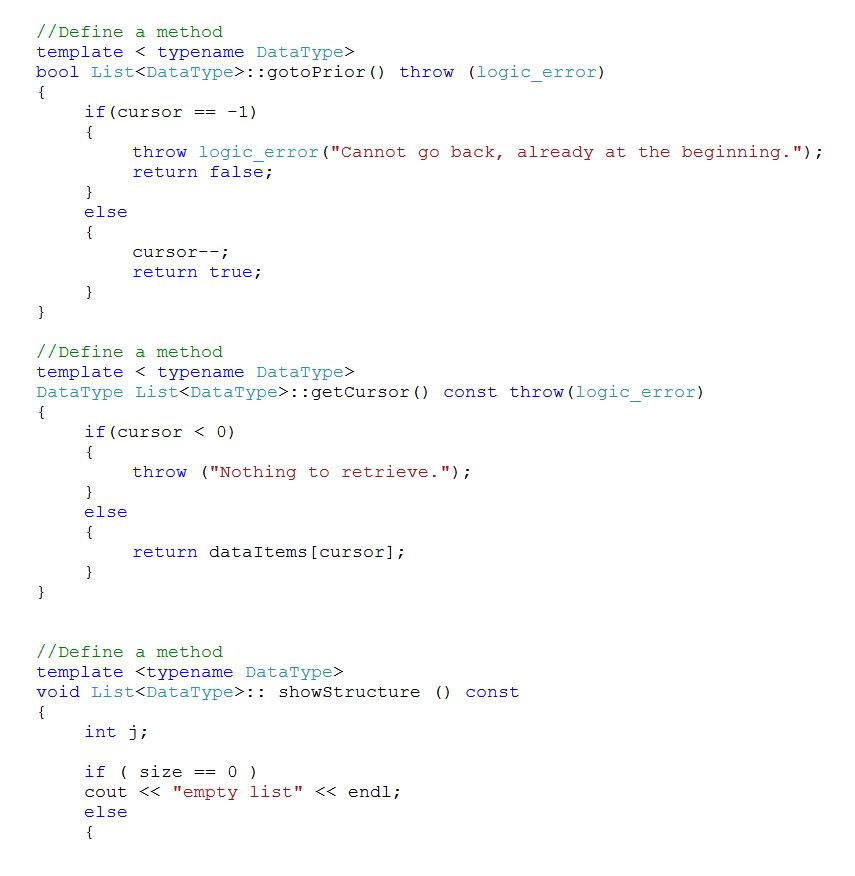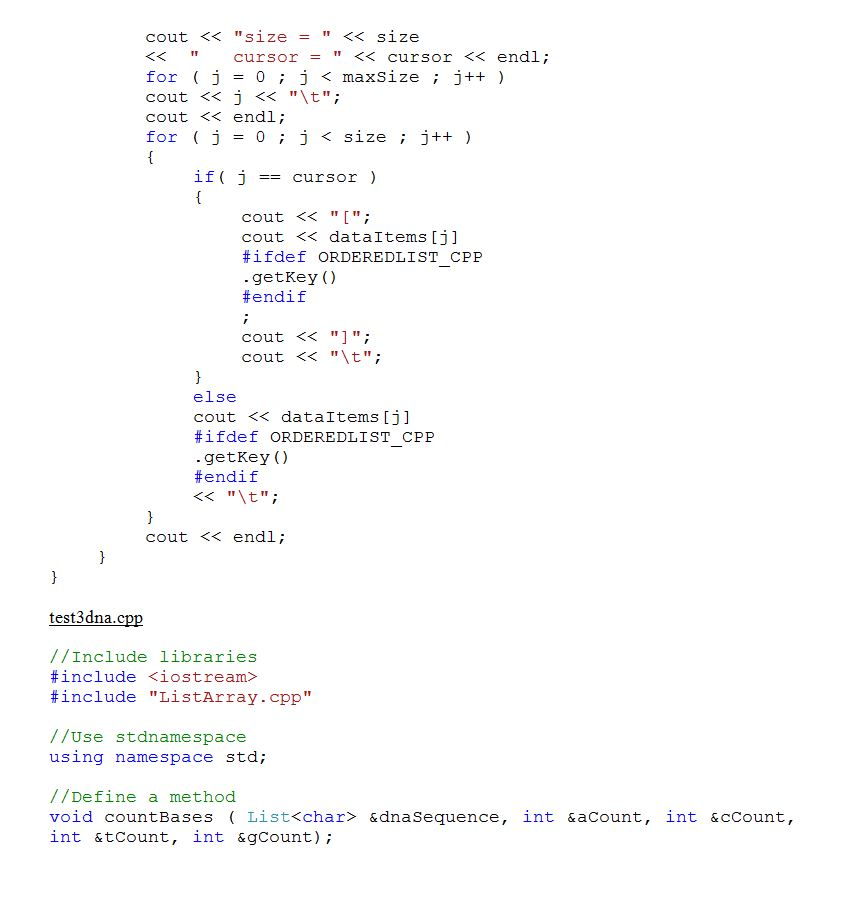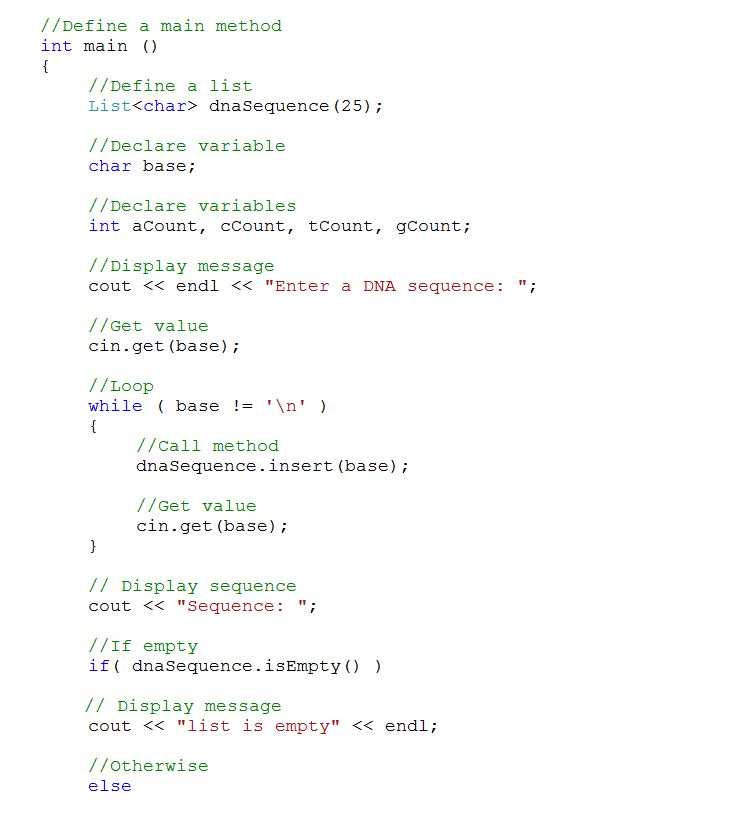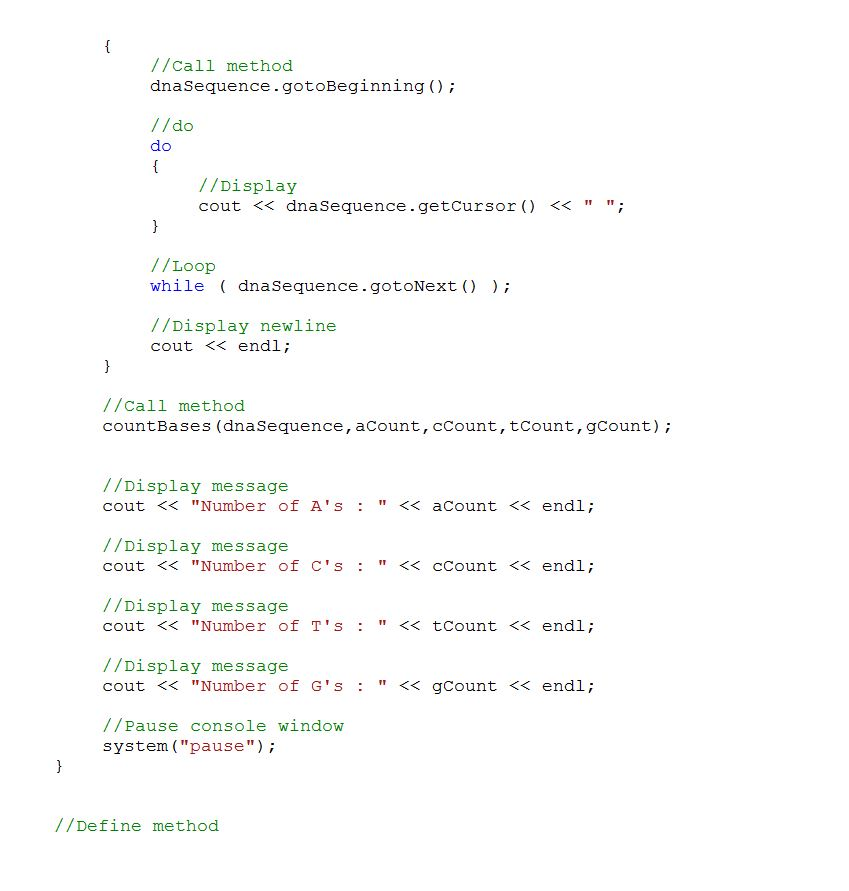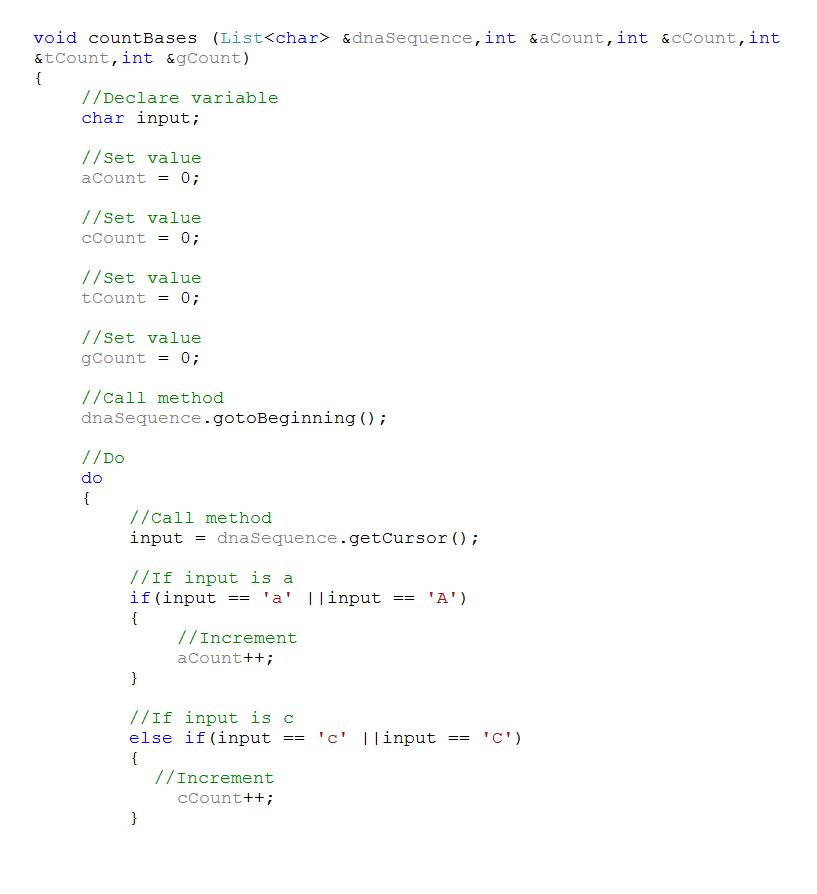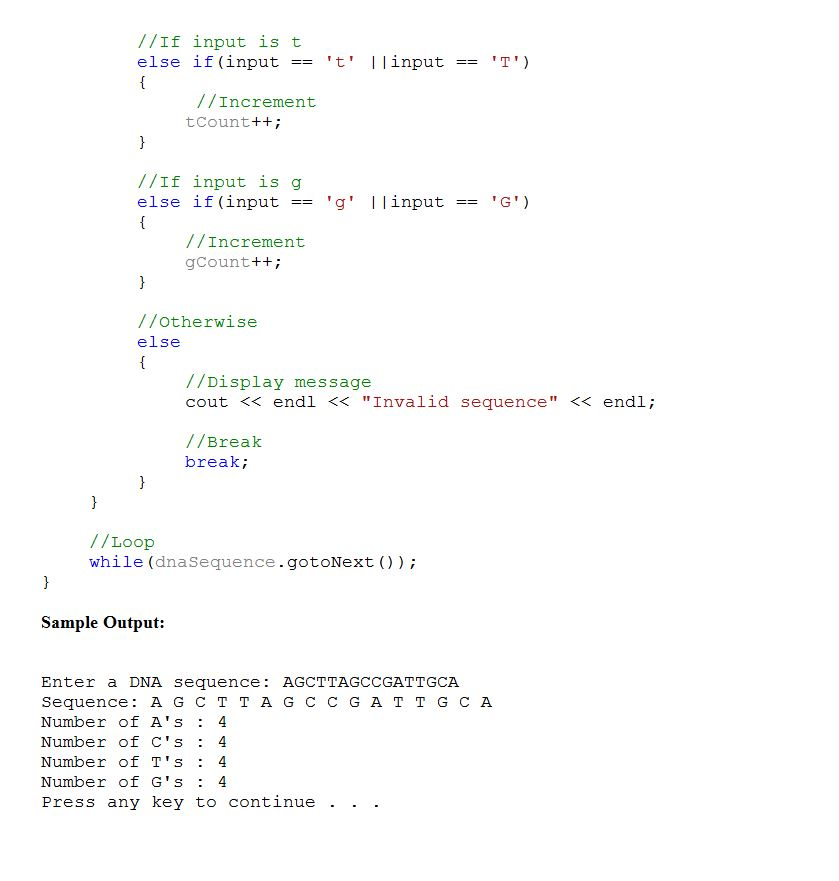Code:

ListArray.h

//include libraries

#ifndef LISTARRAY_H

#define LISTARRAY_H

#include <stdexcept>

#include <iostream>

//Use std namespace

using namespace std;

//Define a class List

template < typename DataType >

class List

{

//Define access specifier

public:

//Declare variable

static const int MAX_LIST_SIZE = 10;

//Define a Constructor

List ( int maxNumber = MAX_LIST_SIZE );

//Define a Constructor

List ( const List& source );

// Define a Constructor

List& operator= ( const List& source );

//Define a Destructor

virtual ~List ();

//Define a method

virtual void insert ( const DataType& newDataItem ) throw ( logic_error );

//Define a method

void remove () throw ( logic_error );

//Define a method

virtual void replace ( const DataType& newDataItem ) throw ( logic_error );

//Define a method

void clear ();

//Define a method

bool isEmpty () const;

//Define a method

bool isFull () const;

//Define a method

void gotoBeginning () throw ( logic_error );

//Define a method

void gotoEnd ()

throw ( logic_error );

//Define a method

bool gotoNext ()

throw ( logic_error );

//Define a method

bool gotoPrior () throw ( logic_error );

//Define a method

DataType getCursor () const throw ( logic_error );

//Define a method

virtual void showStructure () const;

//Define a method

void moveToNth ( int n ) throw ( logic_error );

//Define a method

bool find ( const DataType& searchDataItem ) throw ( logic_error );

//Define access specifier

protected:

//Declare variables

int maxSize, size,cursor;

//Define items

DataType *dataItems;

};

//End

#endif

ListArray.cpp

//Include library

#include “ListArray.h”

//Define a method

template < typename DataType>

List<DataType>::List(int maxNumber)

{

maxSize = maxNumber;

size = 0;

cursor = -1;

dataItems = new DataType[maxSize];

}

//Define a method

template < typename DataType>

List<DataType>::List(const List& source)

{

maxSize = source.maxSize;

size = source.size;

cursor = source.cursor;

dataItems = new DataType[maxSize];

for(int x = 0; x <= source.size; x++)

{

dataItems[x] = source.dataItems[x];

}

}

//Define a method

template < typename DataType>

List<DataType>& List<DataType>::operator= ( const List& source )

{

if(maxSize != source.maxSize)

{

maxSize = source.maxSize;

size = source.size;

for(int x = 0; x <= source.size; x++)

{

dataItems[x] = source.dataItems[x];

}

}

return *this;

}

//Define a method

template < typename DataType>

List<DataType>::~List()

{

maxSize = 0;

size = 0;

cursor = -1;

delete dataItems;

}

//Define a method

template < typename DataType>

void List<DataType>::insert(const DataType& newDataItem) throw (logic_error)

{

if(this->isFull())

{

throw logic_error(“Not Enough Space”);

}

else

{

int cTrack = size – 1;

int mTrack = size;

if(size != 0)

{

for(int x = size; x > cursor + 1; x–)

{

cout << “dataItems[” << mTrack << “] = dataitems[” << cTrack << “];” <<endl;

dataItems[mTrack] = dataItems[cTrack];

cTrack–;

mTrack–;

}

dataItems[cursor + 1] = newDataItem;

}

else

{

dataItems = newDataItem;

}

cursor++;

size++;

}

}

//Define a method

template < typename DataType>

void List<DataType>::remove () throw ( logic_error )

{

if(size == 0)

{

throw logic_error(“Nothing to delete.”);

}

else

{

for(int x = cursor;

x <= size; x++)

{

dataItems[x] = dataItems[x + 1];

}

size–;

if(size == cursor)

{

cursor = 0;

}

}

}

//Define a method

template < typename DataType>

void List<DataType>::replace(const DataType& newDataItem) throw (logic_error)

{

if(size == 0)

{

throw logic_error(“Nothing to replace.”);

}

else

{

dataItems[cursor] = newDataItem;

}

}

//Define a method

template < typename DataType>

void List<DataType>::clear()

{

if(size > 0)

{

int s = size;

for(int x = s; x >= 0; x–)

{

dataItems[x] = 0;

}

size = 0;

cursor = 0;

}

}

//Define a method

template < typename DataType>

bool List<DataType>::isEmpty() const

{

if(size > 0)

{

return false;

}

else

{

return true;

}

}

//Define a method

template < typename DataType>

bool List<DataType>::isFull() const

{

if(size >= maxSize)

{

return true;

}

else

{

return false;

}

}

//Define a method

template < typename DataType>

void List<DataType>::gotoBeginning() throw (logic_error)

{

if(cursor != -1)

{

cursor = 0;

}

else

{

}

}

//Define a method

template < typename DataType>

void List<DataType>::gotoEnd() throw (logic_error)

{

if(size != 0)

{

cursor = size – 1;

}

else

{

}

}

//Define a method

template < typename DataType>

bool List<DataType>::gotoNext() throw (logic_error)

{

if(cursor == size )

{

throw logic_error(“Cannot go to next, already at the end.”);

return false;

}

else if(cursor != size – 1)

{

cursor++;

return true;

}

else

{

return false;

}

}

//Define a method

template < typename DataType>

bool List<DataType>::gotoPrior() throw (logic_error)

{

if(cursor == -1)

{

throw logic_error(“Cannot go back, already at the beginning.”);

return false;

}

else

{

cursor–;

return true;

}

}

//Define a method

template < typename DataType>

DataType List<DataType>::getCursor() const throw(logic_error)

{

if(cursor < 0)

{

throw (“Nothing to retrieve.”);

}

else

{

return dataItems[cursor];

}

}

//Define a method

template <typename DataType>

void List<DataType>:: showStructure () const

{

int j;

if ( size == 0 )

cout << “empty list” << endl;

else

{

cout << “size = ” << size

<< ”   cursor = ” << cursor << endl;

for ( j = 0 ; j < maxSize ; j++ )

cout << j << “t”;

cout << endl;

for ( j = 0 ; j < size ; j++ )

{

if( j == cursor )

{

cout << “[“;

cout << dataItems[j]

#ifdef ORDEREDLIST_CPP

.getKey()

#endif

;

cout << “]”;

cout << “t”;

}

else

cout << dataItems[j]

#ifdef ORDEREDLIST_CPP

.getKey()

#endif

<< “t”;

}

cout << endl;

}

}

test3dna.cpp

//Include libraries

#include <iostream>

#include “ListArray.cpp”

//Use stdnamespace

using namespace std;

//Define a method

void countBases ( List<char> &dnaSequence, int &aCount, int &cCount, int &tCount, int &gCount);

//Define a main method

int main ()

{

//Define a list

List<char> dnaSequence(25);

//Declare variable

char base;

//Declare variables

int aCount, cCount, tCount, gCount;

//Display message

cout << endl << “Enter a DNA sequence: “;

//Get value

cin.get(base);

//Loop

while ( base != ‘n’ )

{

//Call method

dnaSequence.insert(base);

//Get value

cin.get(base);

}

// Display sequence

cout << “Sequence: “;

//If empty

if( dnaSequence.isEmpty() )

// Display message

cout << “list is empty” << endl;

//Otherwise

else

{

//Call method

dnaSequence.gotoBeginning();

//do

do

{

//Display

cout << dnaSequence.getCursor() << ” “;

}

//Loop

while ( dnaSequence.gotoNext() );

//Display newline

cout << endl;

}

//Call method

countBases(dnaSequence,aCount,cCount,tCount,gCount);

//Display message

cout << “Number of A’s : ” << aCount << endl;

//Display message

cout << “Number of C’s : ” << cCount << endl;

//Display message

cout << “Number of T’s : ” << tCount << endl;

//Display message

cout << “Number of G’s : ” << gCount << endl;

//Pause console window

system(“pause”);

}

//Define method

void countBases (List<char> &dnaSequence,int &aCount,int &cCount,int &tCount,int &gCount)

{

//Declare variable

char input;

//Set value

aCount = 0;

//Set value

cCount = 0;

//Set value

tCount = 0;

//Set value

gCount = 0;

//Call method

dnaSequence.gotoBeginning();

//Do

do

{

//Call method

input = dnaSequence.getCursor();

//If input is a

if(input == ‘a’ ||input == ‘A’)

{

//Increment

aCount++;

}

//If input is c

else if(input == ‘c’ ||input == ‘C’)

{

//Increment

cCount++;

}

//If input is t

else if(input == ‘t’ ||input == ‘T’)

{

//Increment

tCount++;

}

//If input is g

else if(input == ‘g’ ||input == ‘G’)

{

//Increment

gCount++;

}

//Otherwise

else

{

//Display message

cout << endl << “Invalid sequence” << endl;

//Break

break;

}

}

//Loop

while(dnaSequence.gotoNext());

}Pages (550 words)
Approximate price: -

Help Me Write My Essay - Reasons:Best Online Essay Writing Service

We strive to give our customers the best online essay writing experience. We Make sure essays are submitted on time and all the instructions are followed.Our Writers are Experienced and Professional

Our essay writing service is founded on professional writers who are on stand by to help you any time.Free Revision Fo all Essays

Sometimes you may require our writers to add on a point to make your essay as customised as possible, we will give you unlimited times to do this. And we will do it for free.Timely Essay(s)

We understand the frustrations that comes with late essays and our writers are extra careful to not violate this term. Our support team is always engauging our writers to help you have your essay ahead of time.Customised Essays &100% Confidential

Our Online writing Service has zero torelance for plagiarised papers. We have plagiarism checking tool that generate plagiarism reports just to make sure you are satisfied.Try it now!

## Calculate the price of your order

Total price:
\$0.00

How it works?Fill in the order form and provide all details of your assignment.Proceed with the payment

Choose the payment system that suits you most.HOW OUR ONLINE ESSAY WRITING SERVICE WORKS

Let us write that nagging essay.By clicking on the "PLACE ORDER" button, tell us your requires. Be precise for an accurate customised essay. You may also upload any reading materials where applicable.Pick A & Writer

Our ordering form will provide you with a list of writers and their feedbacks. At step 2, its time select a writer. Our online agents are on stand by to help you just in case.Editing (OUR PART)

At this stage, our editor will go through your essay and make sure your writer did meet all the instructions.